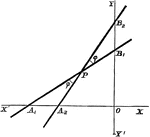### Angle

Finding the angle between two lines whose equations are in intercept form.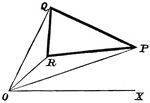### Triangle Area

Finding the area of a triangle in terms of polar coordinates of its three vertices.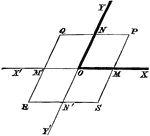### Oblique Axes

Angles on a modified coordinate plane.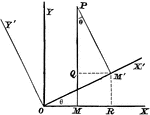### Rectangular Axes

Transforming from one set of rectangular axes to another with the same origin, but different direction.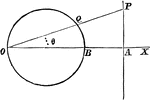### Circle

A circle and triangle situated on coordinate planes.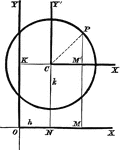### Common Form of Circle

A circle in its common, or central form. This is used to assist students in finding the equation of…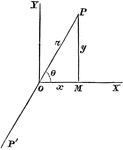### Rectangular Coordinates

Changing from polar to rectangular coordinates.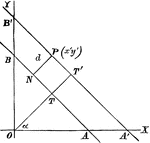### Perpendicular Distance

Finding the perpendicular distance of points whose equation is x cosa + y sina = p.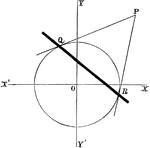### Chord Equation

Tangent lines are drawn from a given external point to a circle. The equation is found by joining their…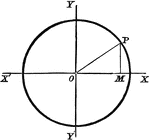### Circle Equation

Finding the equation of a circle with the origin as its center.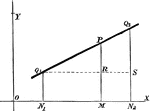### Line Equation

Finding the equation of a line through two given points.### Normal Equation

Finding the equation of the normal at any point on a circle.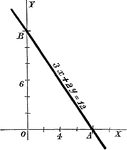### General Form

A line with a standard form equation.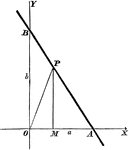### Intercept Form

A line with intercept form equation.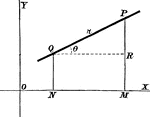### Given Incline

Equation of a line with two given points and a given inclination.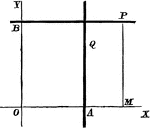### Straight Line

Equation of a straight line: a) parallel to y-axis, b) parallel to x-axis.### Straight Line

A positive slope line making a 60 degree angle with the x-axis.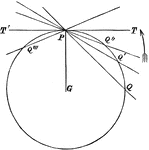### Lines

A circle with many tangent and secant lines passing through it.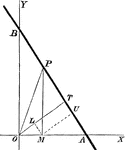### Alternate Method

Another method for using perpendicular form.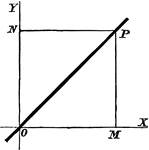### The Origin

A straight line through the origin of a coordinate plane.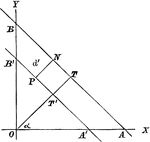### Alternate Form, Perpendicular Distance

An alternate form of the perpendicular distance method.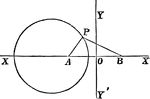### Coordinate Plane

A circle with part of a triangle inscribed in it. They are lying on a coordinate plane.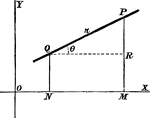### Given Point

Finding the equation of a line drawn through a given point at a given direction.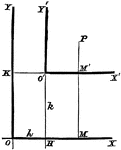### Axes Shift

Any system of coordinate axes to another set which is parallel to the former, but with different origins.